Courses

# The Force Method of Analysis: Beams - 4 Civil Engineering (CE) Notes | EduRev

## Civil Engineering (CE) : The Force Method of Analysis: Beams - 4 Civil Engineering (CE) Notes | EduRev

The document The Force Method of Analysis: Beams - 4 Civil Engineering (CE) Notes | EduRev is a part of the Civil Engineering (CE) Course Structural Analysis.
All you need of Civil Engineering (CE) at this link: Civil Engineering (CE)

Example 9.1

Calculate the support reactions in the continuous beam ABC (see Fig. 9.6a) having constant flexural rigidity EI throughout, due to vertical settlement of the support B by 5 mm as shown in the figure. E = 200 GPa and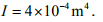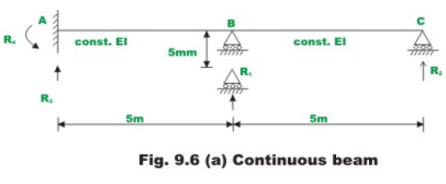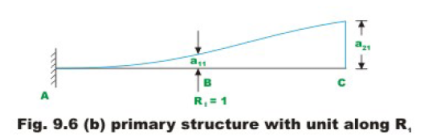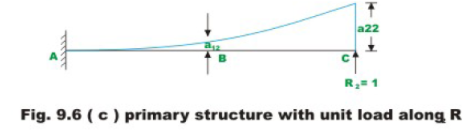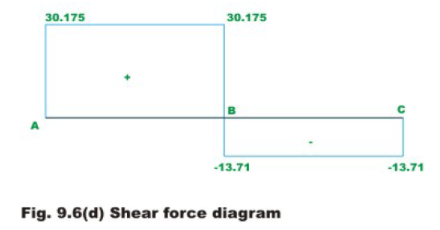As the given beam is statically indeterminate to second degree, choose reaction at B(R1) and C(R2) as the redundants. In this case the cantilever beam AC the basic determinate beam (primary structure). On the determinate beam only redundant reactions are acting. The first column of flexibility matrix is evaluated by first applying unit load along the redundant R1 and determining deflections a11 and a21 respectively as shown in Fig. 9.6b.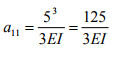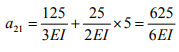(1)

Simply by applying the unit load in the direction of redundant R2, one could evaluate flexibility coefficients a12 and a22 (see Fig. 9.6c).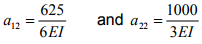(2)

The compatibility condition for the problem may be written as,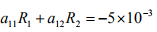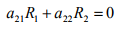(3)

The redundant reactions are,(4)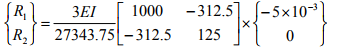(5)

Substituting the values of E and I in the above equation, the redundant reactions are evaluated.

R1 = −43.885 kN and R2 =13.71 kN

R1 acts downwards and R2 acts in the positive direction of the reaction i.e upwards. The remaining two reactions R3 and R4 are evaluated by the equations of equilibrium.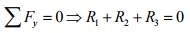Hence R3 = 30.175 kN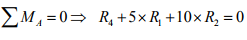Solving for R4 ,

R4 = 82.325 kN.m (counter clockwise)

The shear force and bending moment diagrams are shown in Figs. 9.6d and 9.6e respectively.

Example 9.2

Compute reactions and draw bending moment diagram for the continuous beam ABCD loaded as shown in Fig. 9.7a, due to following support movements.
Support B , 005.0 m vertically downwards.
Support C , 01.0 m vertically downwards.

Assume, E = 200GPa ;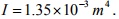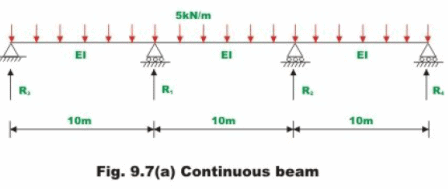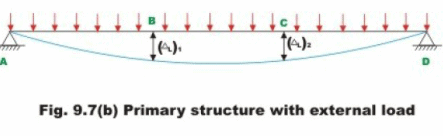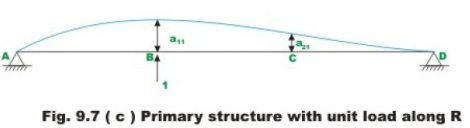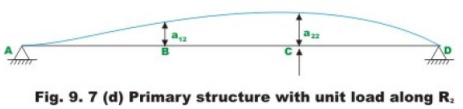The given beam is statically indeterminate to second degree. Select vertical reactions at B(R1) and C(R2) as redundants. The primary structure in this case is a simply supported beam AD as shown in Fig. 9.7b.

The deflection (ΔL)1 and (ΔL)2 of the released structure are evaluated from unit load method. Thus,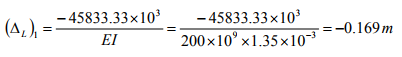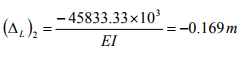(1)

The flexibility matrix is evaluated as explained in the previous example, i.e by first applying unit load corresponding to the redundant and determining deflections a11 and a21 respectively as shown in Fig. 9.7c. Thus,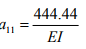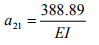(2)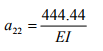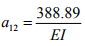In this case the compatibility equations may be written as,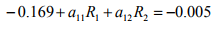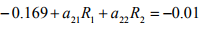(3)

Solving for redundant reactions,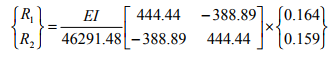(4)

Substituting the value of E and I in the above equation,

R1 = 48.64 kN and R2 = 174.40 kN
Both R1 and R2 acts in the upward direction. The remaining two reactions R3 and R4 are evaluated by the equations of static equilibrium.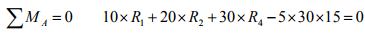Hence R4 = 26.724 kN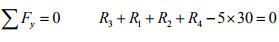Hence  R3 =18.622 kN                             (5)

The shear force and bending moment diagrams are now constructed and are shown in Figs. 9.7e and 9.7f respectively.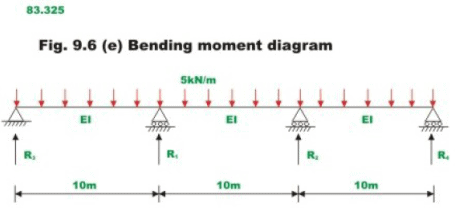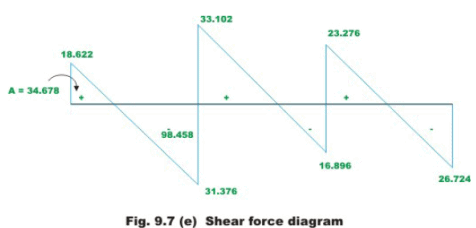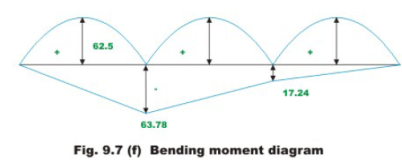Summary

In this lesson, the effect of support settlements on the reactions and stresses in the case of indeterminate structures is discussed. The procedure to calculate additional stresses caused due to yielding of supports is explained with the help of an example. A formula is derived for calculating stresses due to temperature changes in the case of statically indeterminate beams.

Offer running on EduRev: Apply code STAYHOME200 to get INR 200 off on our premium plan EduRev Infinity!

## Structural Analysis

30 videos|122 docs|28 tests

,

,

,

,

,

,

,

,

,

,

,

,

,

,

,

,

,

,

,

,

,

;